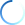1st Edition

# Statistical Inference Based on Divergence Measures

By

## Leandro Pardo

ISBN 9781584886006
Published October 10, 2005 by Chapman and Hall/CRC
512 Pages - 22 B/W Illustrations

USD \$145.00

Prices & shipping based on shipping country

## Book Description

The idea of using functionals of Information Theory, such as entropies or divergences, in statistical inference is not new. However, in spite of the fact that divergence statistics have become a very good alternative to the classical likelihood ratio test and the Pearson-type statistic in discrete models, many statisticians remain unaware of this powerful approach.

Statistical Inference Based on Divergence Measures explores classical problems of statistical inference, such as estimation and hypothesis testing, on the basis of measures of entropy and divergence. The first two chapters form an overview, from a statistical perspective, of the most important measures of entropy and divergence and study their properties. The author then examines the statistical analysis of discrete multivariate data with emphasis is on problems in contingency tables and loglinear models using phi-divergence test statistics as well as minimum phi-divergence estimators. The final chapter looks at testing in general populations, presenting the interesting possibility of introducing alternative test statistics to classical ones like Wald, Rao, and likelihood ratio. Each chapter concludes with exercises that clarify the theoretical results and present additional results that complement the main discussions.

Clear, comprehensive, and logically developed, this book offers a unique opportunity to gain not only a new perspective on some standard statistics problems, but the tools to put it into practice.

DIVERGENCE MEASURES: DEFINITION AND PROPERTIES
Introduction
Phi-divergence. Measures between Two Probability Distributions: Definition and Properties
Other Divergence Measures between Two Probability Distributions
Divergence among k Populations
Phi-disparities
Exercises

ENTROPY AS A MEASURE OF DIVERSITY: SAMPLING DISTRIBUTIONS
Introduction
Phi-entropies. Asymptotic Distribution
Testing and Confidence Intervals for Phi-entropies
Multinomial Populations: Asymptotic Distributions
Maximum Entropy Principle and Statistical Inference on Condensed Ordered Data
Exercises

GOODNESS-OF-FIT: SIMPLE NULL HYPOTHESIS
Introduction
Phi-divergences and Goodness-of-fit with Fixed Number of Classes
Phi-divergence Test Statistics under Sparseness Assumptions
Nonstandard Problems: Tests Statistics based on Phi-divergences
Exercises

OPTIMALITY OF PHI-DIVERGENCE TEST STATISTICS IN GOODNESS-OF-FIT
Introduction
Asymptotic Effciency
Exact and Asymptotic Moments: Comparison
A Second Order Approximation to the Exact Distribution
Exact Powers Based on Exact Critical Regions
Small Sample Comparisons for the Phi-divergence Test Statistics
Exercises

MINIMUM PHI-DIVERGENCE ESTIMATORS
Introduction
Maximum Likelihood and Minimum Phi-divergence Estimators
Properties of the Minimum Phi-divergence Estimator
Normal Mixtures: Minimum Phi-divergence Estimator
Minimum Phi-divergence Estimator with Constraints: Properties
Exercises

GOODNESS-OF-FIT: COMPOSITE NULL HYPOTHESIS
Introduction
Asymptotic Distribution with Fixed Number of Classes
Nonstandard Problems: Test Statistics Based on Phi-divergences
Exercises
Testing Loglinear Models Using Phi-divergence Test Statistics
Introduction
Loglinear Models: Definition
Asymptotic Results for Minimum Phi-divergence Estimators in Loglinear Models
Testing in Loglinear Models
Simulation Study
Exercises

PHI-DIVERGENCE MEASURES IN CONTINGENCY TABLES
Introduction
Independence
Symmetry
Marginal Homogeneity
Quasi-symmetry
Homogeneity
Exercises

TESTING IN GENERAL POPULATIONS
Introduction
Simple Null Hypotheses: Wald, Rao, Wilks and Phi-divergence Test Statistics
Composite Null Hypothesis
Multi-sample Problem
Some Topics in Multivariate Analysis
Exercises